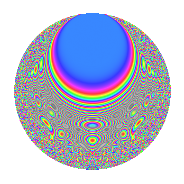# Properties

 Label 2541.2.cLevel 2541 Weight 2 Character orbit c Rep. character $$\chi_{2541}(1693,\cdot)$$ Character field $$\Q$$ Dimension 144 Sturm bound 704

# Related objects

## Defining parameters

 Level: $$N$$ = $$2541 = 3 \cdot 7 \cdot 11^{2}$$ Weight: $$k$$ = $$2$$ Character orbit: $$[\chi]$$ = 2541.c (of order $$2$$ and degree $$1$$) Character conductor: $$\operatorname{cond}(\chi)$$ = $$77$$ Character field: $$\Q$$ Sturm bound: $$704$$

## Dimensions

The following table gives the dimensions of various subspaces of $$M_{2}(2541, [\chi])$$.

Total New Old
Modular forms 376 144 232
Cusp forms 328 144 184
Eisenstein series 48 0 48

## Trace form

 $$144q - 148q^{4} - 144q^{9} + O(q^{10})$$ $$144q - 148q^{4} - 144q^{9} + 8q^{15} + 180q^{16} - 24q^{23} - 136q^{25} + 148q^{36} - 16q^{37} - 8q^{42} - 8q^{49} - 16q^{53} + 32q^{56} + 16q^{58} - 48q^{60} - 292q^{64} + 40q^{67} - 20q^{70} + 80q^{71} + 144q^{81} - 48q^{86} - 60q^{91} + 128q^{92} - 32q^{93} + O(q^{100})$$

## Decomposition of $$S_{2}^{\mathrm{new}}(2541, [\chi])$$ into newform subspaces

The newforms in this space have not yet been added to the LMFDB.

## Decomposition of $$S_{2}^{\mathrm{old}}(2541, [\chi])$$ into lower level spaces

$$S_{2}^{\mathrm{old}}(2541, [\chi]) \cong$$ $$S_{2}^{\mathrm{new}}(77, [\chi])$$$$^{\oplus 4}$$$$\oplus$$$$S_{2}^{\mathrm{new}}(231, [\chi])$$$$^{\oplus 2}$$$$\oplus$$$$S_{2}^{\mathrm{new}}(847, [\chi])$$$$^{\oplus 2}$$

## Hecke Characteristic Polynomials

There are no characteristic polynomials of Hecke operators in the database